## Looking for your content...Type it here

### GATE Previous Years Papers on Instrumentation Engineering

Q.1 – Q.20 carry one mark each

1) You have gone to a cyber-cafe with a friend. You found that the cyber-café has only three terminals. All terminals are unoccupied. You and your friend have to make a random choice of selecting a terminal. What is the probability that both of you will NOT select the same terminal?

(A)
(B)
(C)
(D)1

2) Probability density function p(x) of a random variable x is as shown below. The value of α is(A) c2
(B) c1
(C) c)(b2+
(D) c)(

3) A linear ordinary differential equation is given as

where δ (t) is an impulse input. The solution is found by Euler’s forward-difference method that uses an integration step h. What is a suitable value of h?
(A) 2.0
(B) 1.5
(C) 1.0
(D) 0.2

4) A 4 kΩ, 0.02 W potentiometer is used in the circuit shown below. The minimum value of the resistance Rs in order to protect the potentiometer is(A) 2.23kΩ
(B) 2.71kΩ
(C) 3.82kΩ
(D) 8.92kΩ

5) The root-mean-square value of a voltage waveform consisting of a superimposition of 2 V dc and a 4 V peak-to-peak square wave is
(A) 2 V
(B) V6
(C) V8
(D) V12

6) “Vena Contracta” is the cross section where the flow area is a minimum for a restriction type flowmeter. For an orifice meter, if d is the diameter of the orifice opening, then the area of the vena contracta is approximately
(A) 42dπ
(B) 499.02dπ
(C) 48.02dπ
(D) 46.02

7) A type J (iron-constantan) thermocouple has a voltage sensitivity of 55 μ V/°C
A digital voltmeter (DVM) is used to measure the voltage under the condition shown in the following figure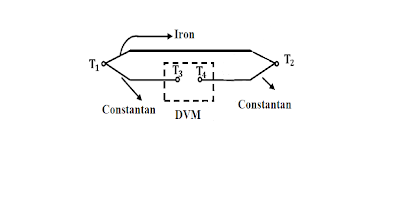Given that T1 = 300°C, T2 = 100° C and T3 = T4= 20° C, the meter will indicate a voltage of
(A) 11.0 m V
(B) 15.4 m V
(C) 16.5 m V
(D) 17.6 m V

8) If the value of the resistance R in the following figure is increased by 50%, then voltage gain of the amplifier shown in the figure will change by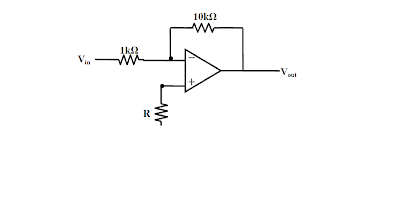(A)50%
(B) 5%
(C) 50%
(D)negligible amount

9) When the switch S2 is closed the gain of the programmable gain amplifier shown in the following Figure is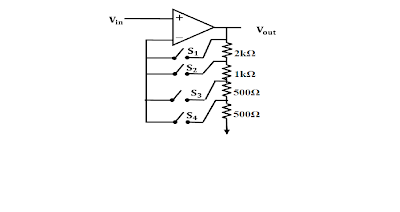(A) 0.5
(B) 2
(C) 4
(D)8

10) A memory mapped I/O device has an address of 00F0H. Which of the following 8085 instructions outputs the content of the accumulat or to the I/O device?
(A)LXI H, 00F0H
MOV M, A
(B) LXI H, 00F0H
OUT M
(C) LXI H, 00F0H
OUT F0H
(D)LXI H, 00F0H
MOV A, M

11) A voltmeter has a range of [4 V, 20 V] and a resolution of 1 mV. The dynamic range is

(A)24 dB
(B) 60 dB
(C) 72 dB
(D)84 dB

12) 12. A shielded cable is used for signal transmission between two instruments kept some distance apart. The shield should be

(A)Left open at both ends
(B)Grounded at one end
(C)Grounded at both ends
(D)Connected to ground at both ends using a resistance equal to the characteristic resistance of the cable

13) Compared to a conventional two-phase induction motor, a two-phase servomotor has

(A)A smaller XRratio and a lighter rotor
(B) the same XRratio and a heavier rotor
(C) a larger XRratio and a heavier rotor
(D) a larger XRratio and a lighter rotor

14) The state-variable representation of a plant is given by
x = Ax + Bu
y = Cx.
where x is the state, u is the input and y is the output. Assuming zero initial condition, the impulse response of the plant is given by
(A) exp (At)
(B) ∫ exp [A (t τ)] Bu (τ) dτ
(C) C exp (At) B
(D)C ∫ exp [A (t τ)] Bu (τ) dτ

15) Correct input-output characteristic curve of a semiconductor laser is16) In a fiber optic displacement sensor shown in the following figure, the ratio of the output light intensity DOES NOT depend on(A)Numerical aperture of the fibers
(B) Length L of the fibers
(C)Distance d
(D)Reflectivity of the mirror

17) For bio-potential recording, the impedance of an Ag/AgCl electrode, as compared to that of an Ag electrode of the same size, is

(A) lower at all frequencies
(B) lower for frequencies less than 10 Hz and higher for frequencies greater than 300 Hz
(C) higher for frequencies less than 10 Hz and lower for frequencies greater than 300 Hz
(D) higher at all frequencies

18) Collimated light beams from a HeNe laser and a Sodium vapour lamp (used for street lighting) are focused using a lens. The size of the spot at the focal point due to the laser is relatively smaller because

(A) laser light is relatively more monochromatic
(B) the limiting divergence of laser light is relatively smaller
(C) the output power of the laser is relatively larger
(D) the wavelength of the laser is relatively longer

19) 19. An X-ray source radiates two characteristic wavelengths : λ1 and λ2, where λ1 < λ2
The shorter wavelength can be filtered out by passing the radiation through .
(A) a narrow slit
(B) an interference filter
(C) a crystalline material whose absorption edge lies between λ1 and λ2
(D) a polarizer that rotates the polarization state of radiation at λ2

20. A Pirani gage measures vacuum pressure and works on the principle of

(A) change in ionizing potential
(B) change in thermal conductivity
(C) deformation of elastic body
(D) change in self-inductance

Q.21 to Q.75 carry two marks each

21. Two dices are rolled simultaneously. The probability that the sum of digits on the top surface of the two dices is even, is
(A) 0.5
(B) 0.25
(C) 0.167
(D) 0.125

22). The plot of a function f(x) is shown in the following figure. A possible expression for the function f(x) is
(A) ()xexp
(B) −x1exp
(C) ()xexp−
(D)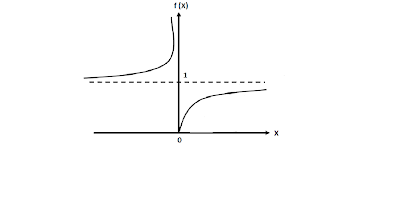23. For a given 2 x 2 matrix A, it is observed that

24. For an initial value problem and. Various solutions are written in the following groups. Match the type of solution with the correct expression.

 Group 1 Group 2 P. General solution of homogeneous equations 1. 0.1ex Q. Particular integral 2. e-x (A cos 10 x + B sin 10 x) R. Total solution satisfying boundary conditions 3. (A cos 10 x + B sin 10 x)

(A)P2, Q1, R3
(B) P1, Q3, R2
(C) P1, Q2, R3
(D)P3, Q2, R1

25. The value of the integral of the complex function

Along the path |s| = 3 is
(A)2πj
(B) 4πj
(C) 6πj
(D)8πj

26. For k = 0, 1, 2, …………., the steps of Newton-Raphson method for solving a non-linear equation is given as

Starting from a suitable initial choice as k tends to ∞, the iterate xk

(A) 1.7099 tends to
(B) 2.2361
(C) 3.1251
(D) 5.0000

27) The function y = sin φ, (φ > 0) is approximated as y = φ, where φ is in radian. The maximum value of φ for which the error due to the approximation is within ± 2% is

28. A certain quantity x is calculated from measured values of a, b, and c using the formula,

where k is a constant. The maximum limiting error in each of the three measured quantities

is Δ. The maximum limiting error in x will be

29)In the circuit shown in the following figure, the input voltage vi(t) is constant at 2 V for time >> 1 s and then it changes to 1 V. The output voltage, v0(t), 2 s after the change will be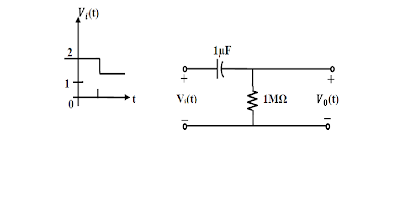(A)– exp (2) V
(B) – 1 + exp (2) V
(C) exp (2) V
(D)1 – exp (2) V

30) A metal wire has uniform cross-section A, length , and resistance R between its two end points. It is uniformly stretched so that its length becomes . The new resistance is

(A) α R
(B) α2
(C) Rα
(D) eα

31. The secondary induced voltages of a LVDT, shown in the following figure, at null position are = 1.0 V ∠0°, = 1.0 V ∠10° respectively. Then the null voltage of the LVDT is(A)0 V
(B) 0.014 V
(C) 0.174 V
(D)2 V

32. A variable air gap type capacitor consists of two parallel plates: a fixed plate and a moving plate at a distance x. If a potential V is applied across the two plates, then force of attraction between the (A)F ∝ x
(B)
2
2x1F∝
(C) x1F∝
(D)Fplates is related to x as

33.An accelerometer has a seismic mass of 100 microgram and natural frequency of 1 kHz. With g the acceleration due to gravity, the static sensitivity of the accelerometer in (nm/g) is
(A)2.58 nm/g
(B) 9.81 nm/g
(C) 7.28 × 102
(D) 6.28 × nm/g

34) In the circuit shown in the following figure, the op amp has input bias current Ib <10 nA, and input offset voltage Vio < 1 mV. The maximum dc error in the output voltage is(A) 1.0 mV
(B) 2.0 mV
(C) 2.5 mV
(D) 3.0 mV

35. The potential difference between the input terminals of an op amp may be treated to be nearly zero, if

(A)The two supply voltages are balanced
(B) The output voltage is not saturated
(C) The op amp is used in a circuit having negative feedback
(D)There is a dc bias path between each of the input terminals and the circuit ground

36. A dual op amp instrumentation amplifier is shown below. The expression for the output of the amplifier is given by37. An amplifier circuit is shown below. Assume that the transistor works in active region. The low frequency small-signal parameters for the transistor are gm = 20 mS, β0 = 50, =∞, rb =0. What is the voltage gain,(A) 0.967
(B) 0.976
(C) 0.983
(D) 0.998

38. The biasing circuit of a silicon transistor is shown below. If β = 80, then what is VCE for the transistor ?